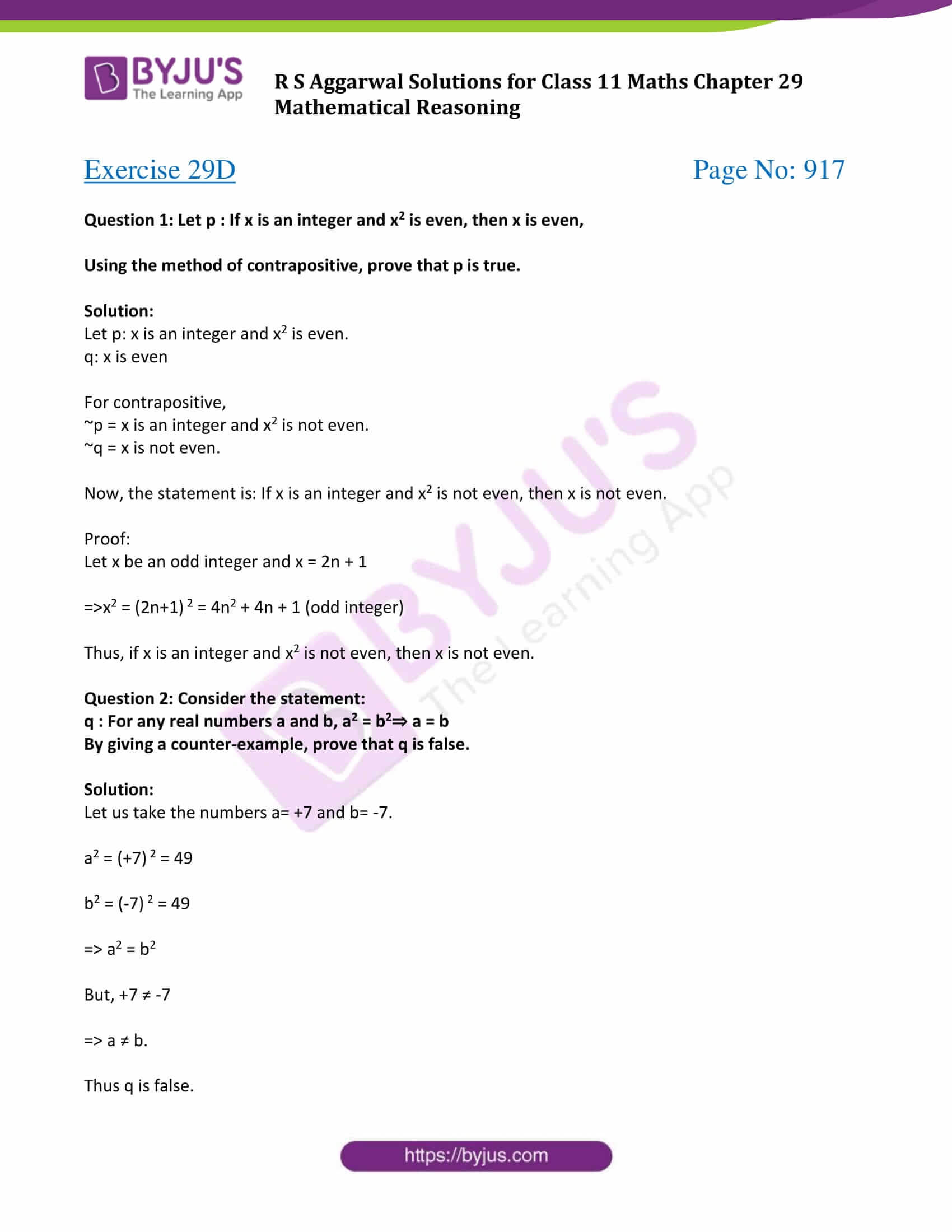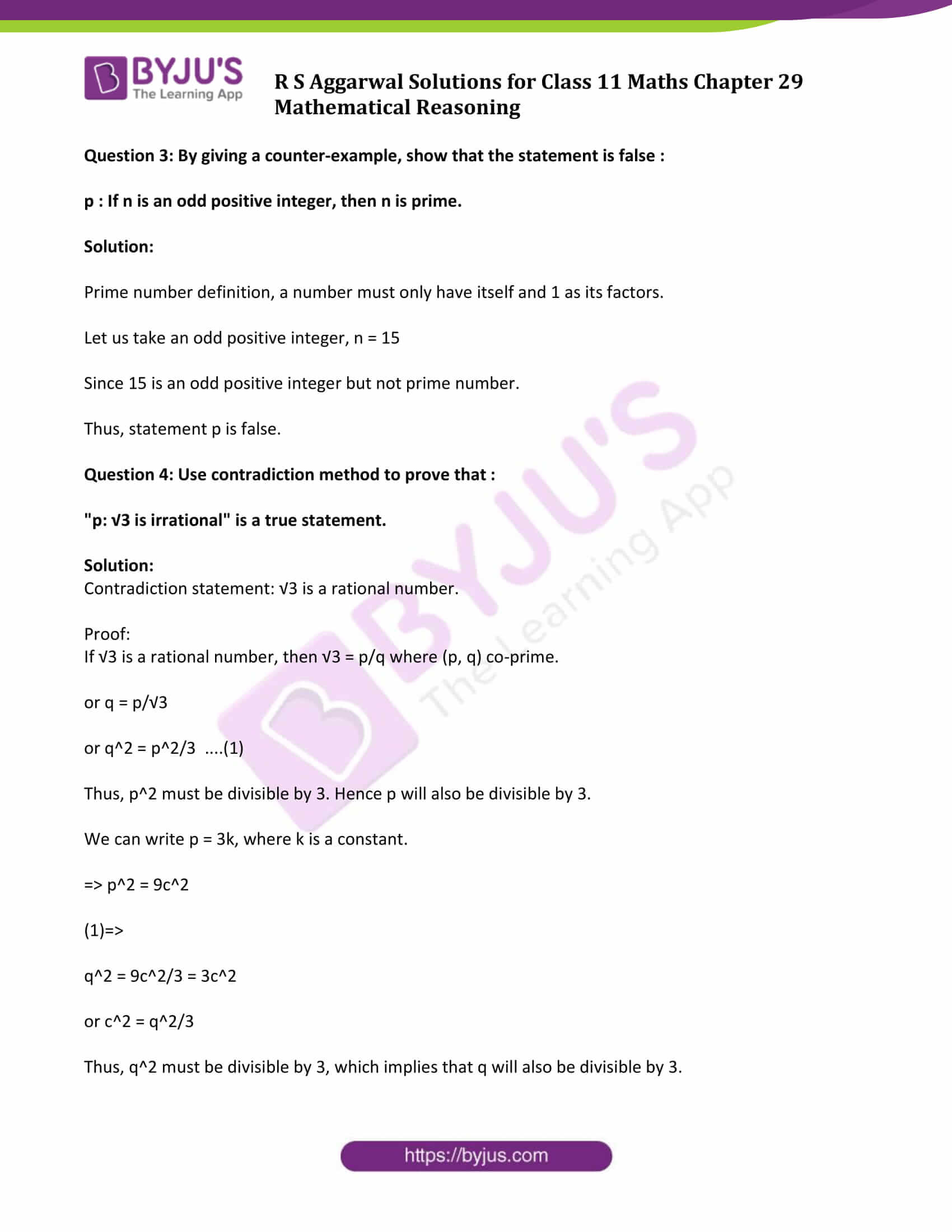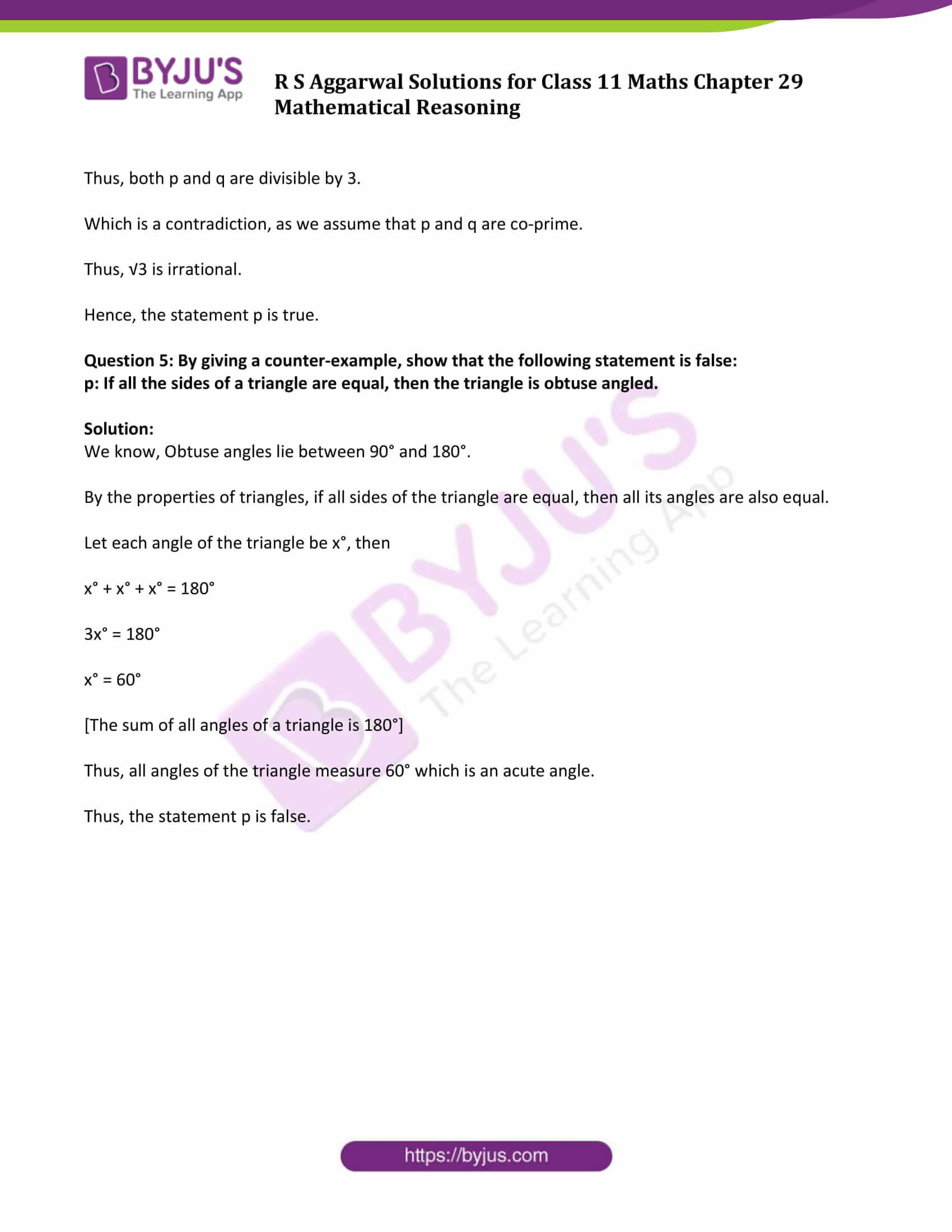# R S Aggarwal Solutions for Class 11 Maths Chapter 29 Mathematical Reasoning Exercise 29D

The R S Aggarwal Class 11 solutions for the chapter Mathematical Reasoning exercise 29D is given here. This exercise is based on validating statements and proving results by contradiction. Students have to follow some general rules for checking whether a given compound statement is true or not. Also, in order to check whether a given statement p is true, we assume that p is false, i.e. ~p is true. R S Aggarwal class 11 exercise 29D pdf contains solution of all the questions listed in this exercise.

## Download PDF of R S Aggarwal Solutions for Class 11 Maths Chapter 29 Mathematical Reasoning Exercise 29D### Access Answers to Maths R S Aggarwal Class 11 Chapter 29 Mathematical Reasoning Exercise 29D Page number 917

Question 1: Let p : If x is an integer and x2 is even, then x is even,

Using the method of contrapositive, prove that p is true.

Solution:

Let p: x is an integer and x2 is even.

q: x is even

For contrapositive,

~p = x is an integer and x2 is not even.

~q = x is not even.

Now, the statement is: If x is an integer and x2 is not even, then x is not even.

Proof:

Let x be an odd integer and x = 2n + 1

⇒x2 = (2n+1) 2 = 4n2 + 4n + 1 (odd integer)

Thus, if x is an integer and x2 is not even, then x is not even.

Question 2: Consider the statement:

q : For any real numbers a and b, a2 = b2⇒ a = b

By giving a counter-example, prove that q is false.

Solution:

Let us take the numbers a= +7 and b= -7.

a2 = (+7) 2 = 49

b2 = (-7) 2 = 49

⇒ a2 = b2

But, +7 ≠ -7

⇒ a ≠ b.

Thus q is false.

Question 3: By giving a counter-example, show that the statement is false :

p : If n is an odd positive integer, then n is prime.

Solution:

Prime number definition, a number must only have itself and 1 as its factors.

Let us take an odd positive integer, n = 15

Since 15 is an odd positive integer but not prime number.

Thus, statement p is false.

Question 4: Use contradiction method to prove that :

“p: √3 is irrational” is a true statement.

Solution:

Contradiction statement: √3 is a rational number.

Proof:

If √3 is a rational number, then √3 = p/q where (p, q) co-prime.

or q = p/√3

or q^2 = p^2/3 ….(1)

Thus, p^2 must be divisible by 3. Hence p will also be divisible by 3.

We can write p = 3k, where k is a constant.

⇒ p^2 = 9c^2

(1)⇒

q^2 = 9c^2/3 = 3c^2

or c^2 = q^2/3

Thus, q^2 must be divisible by 3, which implies that q will also be divisible by 3.

Thus, both p and q are divisible by 3.

Which is a contradiction, as we assume that p and q are co-prime.

Thus, √3 is irrational.

Hence, the statement p is true.

Question 5: By giving a counter-example, show that the following statement is false:

p: If all the sides of a triangle are equal, then the triangle is obtuse angled.

Solution:

We know, Obtuse angles lie between 90° and 180°.

By the properties of triangles, if all sides of the triangle are equal, then all its angles are also equal.

Let each angle of the triangle be x°, then

x° + x° + x° = 180°

3x° = 180°

x° = 60°

[The sum of all angles of a triangle is 180°]

Thus, all angles of the triangle measure 60° which is an acute angle.

Thus, the statement p is false.

## Access other exercise solutions of Class 11 Maths Chapter 29 Mathematical Reasoning

Exercise 29A Solutions

Exercise 29B Solutions

Exercise 29C Solutions

## R S Aggarwal Solutions for Class 11 Maths Chapter 29 Exercise 29D

Class 11 Maths Chapter 29 Mathematical Reasoning Exercise 29D is based on the following topics:

• Validating Statements

-Direct method

-Contrapositive method